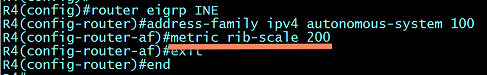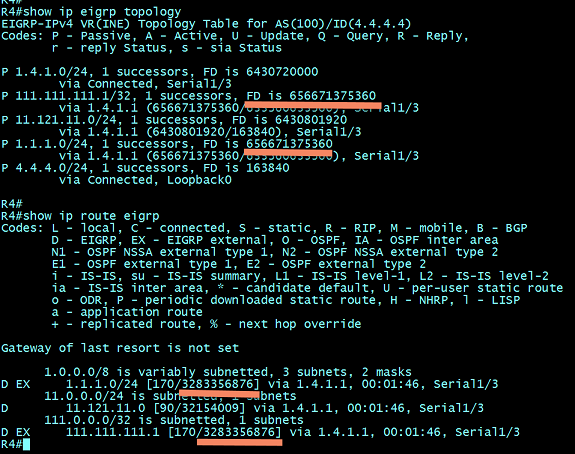blog
Understanding the EIGRP c ...
31 July 18

## Understanding the EIGRP command, "metric rib-scale"

Posted byKeith BogartYou may recall that, when using Named-Mode EIGRP configuration you have automatic access to EIGRP Wide Metrics.  In addition to providing you a new K-Value (K6 which is used against Jitter and Energy) the EIGRP Distance formula has been revised (what they call, "scaled") to account for links above-and-beyond 10Gbps.  Remember that with Classic-Mode EIGRP, the formula looked like this:

metric = ([K1 * bandwidth + (K2 * bandwidth) / (256 - load) + K3 * delay] * [K5 / (reliability + K4)]) * 256

In the formula, the "**bandwidth**" value was represented as:

BW = 10^7 / minimum BW

In the formula above, the "minimum BW" was represented as Kbps. The problem with this "classic" method was all links with a bandwidth higher than 10Gbps (10,000,000,000 bps, represented as 10,000,000 Kbps in the formula) were given the same BW value as 10Gbps.  In other words, whether you put a single link of 10Gbps into that formula,  a link of 40Gbps, or an Etherchannel with a combined bandwidth of 80Gbps...they all equated to "1". So in Classic Mode EIGRP, EIGRP couldn't distinguish between these types of links to develop an accurate path to a destination.

When EIGRP Wide-Metrics were developed, Cisco applied an "EIGRP_WIDE_SCALE" factor against some portions of the formula (which equates to the value of 65,536) to account for faster links (as well as smaller delay values).  They also changed the terminology in the formula from "bandwidth" to "throughput". So now the "new" formula for EIGRP Wide-Metrics does the following to the "minimum bandwidth" portion of the formula:

Minimum Throughput = (10^7 * 65536)/Bw), (remember that Bw is in Kbps) where 65536 is the "EIGRP_WIDE_SCALE" constant.

By multiplying 10^7 against 65,536 EIGRP, Wide-Metrics can now accurately differentiate between links of any speed/bandwidth. EIGRP Wide-Metrics also multiply this value of 65,536 (the "EIGRP_WIDE_SCALE" constant) against the Delay sum.

But here's the problem,  the computed value of this new formula might NOT FIT into the IP Routing Table (called the "RIB" - Routing Information Base).

When you view the output of "show ip route" for any given route, you see two values contained in brackets.  For an EIGRP-learned route, the first number in the brackets represents the Administrative Distance.  The second value represents what I call the "EIGRP Distance".  Others call this simply the route "metric" or "EIGRP Composite Cost".  No matter what term you use, this field in the RIB is only 4-bytes long.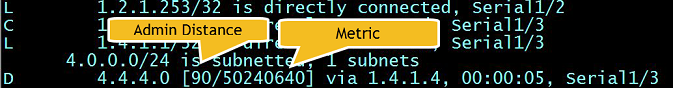Here is the problem,  EIGRP wide metrics (because they have an "EIGRP_WIDE_SCALE" multiplier of 65,536 used against several of the vector-metrics such as bandwidth and delay) could come up with a distance value so large...that the resulting distance value doesn't FIT within a 4-byte value in the RIB.

The maximum decimal value that can be contained within a 4-byte number is 4,294,967,296.  However, if you were to place one's (1's) in each placeholder the EIGRP wide-metrics formula, the resultant bandwidth value (by itself) would be so large that it would break the boundaries of a 4-byte placeholder in the RIB:

BW = (10^7 * 65536)/1) = 655,360,000,000

and that is even BEFORE adding the sum-of-the-delays into the mix.:

((K1*[655,360,000,000) + (K2*Scaled Bw)/(256 – Load) + (K3*Scaled Delay)*(K5/(Reliability + K4)))

The result would be, that while EIGRP was able to calculate a Distance value, that value would be too large to be placed into the RIB. This could happen in a couple of scenarios:

• An EIGRP packet containing a really slow-speed link in the path (like a 56Kbps dialup link)
• Redistribution of other protocols into EIGRP, and selecting a "bandwidth" value (within the "metric" keyword) that was too low.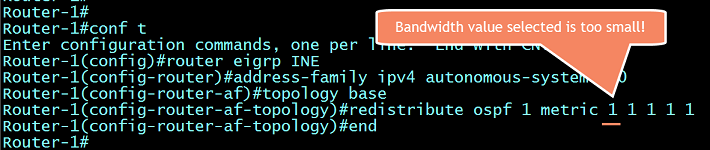And so here's the rub...EIGRP Wide-Metrics supply the ability to differentiate between links of all kinds of different bandwidth values (due to the additional "EIGRP_WIDE_SCALE" factor of 65,536) but the resultant EIGRP Distance value could be too large to fit into the 4-byte "Metric" field within the RIB. If that were the case, this is what you'd see (notice the words, "FD is Infinity" below for the EIGRP routes to 111.111.111.1/32 as well as 1.1.1.0/24)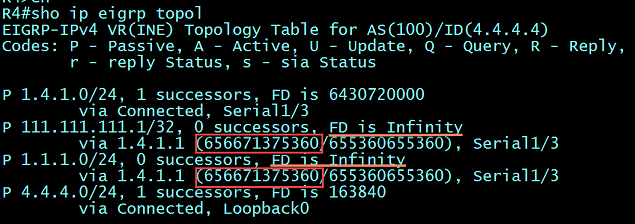Well...those engineers at Cisco were pretty smart and incorporated a special little "tweak" into Wide-Metrics to account for just this problem. This tweak is called the "metric rib-scale". What this does, is to take all EIGRP Feasible Distance values (which may-or-may-not be too large to fit into the 4-byte RIB "metric" value) and DIVIDE THEM by a value called...you guessed it, the "metric rib-scale". The default value of the "metric rib-scale" is 128 which, for most normal routes, is enough to bring them down to size to fit into the RIB. This value can be seen in the following output: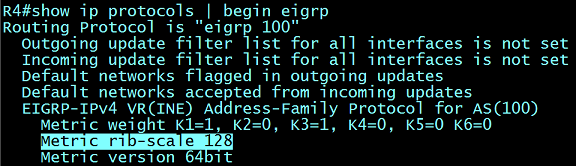This explains why, when viewing the EIGRP Topology Table, an entry for a prefix will display both the 64-bit EIGRP Distance value...as well as the "scaled" values (that was divided by 128) as the "RIB" value: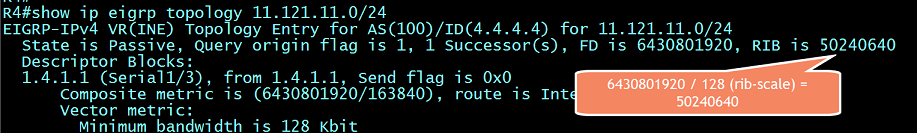And here you can see that scaled RIB metric reflected in the IP Routing Table (since the original EIGRP Feasible Distance was too large to fit):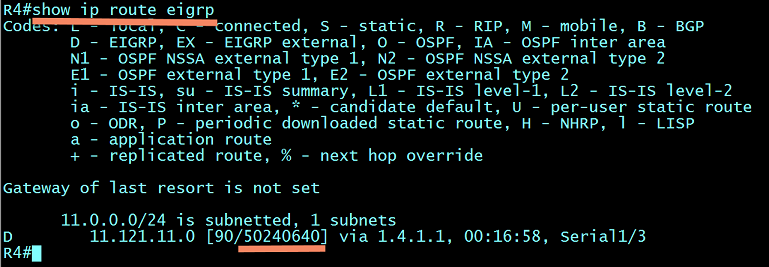But sometimes, the 64-bit Feasible Distance of a route is so large, that scaling/dividing it by the default RIB-Scale value of 128 simply isn't enough. As I previously showed you, these types of EIGRP Topology entries will show as "FD is Infinity". It is for this reason, that one may need to adjust this value to a larger RIB-Scale factor (using the EIGRP command, "metric rib-scale") such that the resulting quotient is small enough to fit into the RIB.

For example, let's take a look at this output again...Even if we divide the FD of 656,671,375,360 by the default RIB-Scale value of 128 the quotient would be 5,130,245,120 which is still larger than our maximum allowable RIB metric of 4,294,967,296. It is for this reason that we would need to adjust the RIB-Scale value to something else (larger than 128) to create a quotient that was smaller than 4,294,967,296. The RIB-Scale is a configurable number between "1" and "255". So by increasing the number beyond the default of 128 we can create quotients that are small enough to fit within the RIB (IP Routing Table).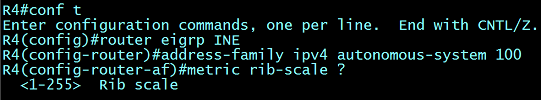So let's apply a new RIB-Scale value to EIGRP and see how that same route, which was previously listed as "Infinity" can fit into the RIB;

(BEFORE...with the default RIB-Scale value)(AFTER applying a larger RIB-Scale value)